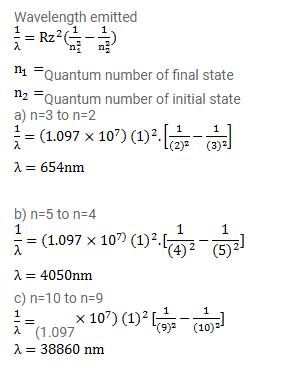# Find the wavelength of the radiation emitted by hydrogen in the transitions

Question:

Find the wavelength of the radiation emitted by hydrogen in the transitions

(a) $n=3$ to $n=2$,

(b) $n=5$ to $n=4$,

(c) $n=10$ to $n=9$.

Solution: